# AP Physics 1 Question 36: Answer and Explanation

### Test Information

Question: 36

6.A tube with one end closed and one end open resonates for a wave with wavelength λa as shown. The next shorter wavelength at which resonance will occur is λb. The ratio of these two wavelengths λab is

• A.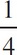• B.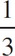• C.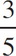• D.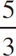D Resonance occurs at λ =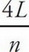for odd integers. This means at 4L,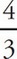L,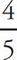L,…. We are shown a picture where a wave is in a tube of length L. This means λa =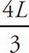. The next shorter wavelength is λb =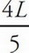. The ratio of these is or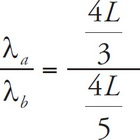or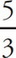.